# RS Aggarwal Class 8 Solutions Chapter 2 Exponents Ex 2A

## RS Aggarwal Class 8 Solutions Chapter 2 Exponents Ex 2A3

These Solutions are part of RS Aggarwal Solutions Class 8. Here we have given RS Aggarwal Solutions Class 8 Chapter 2 Exponents Ex 2A.

Other Exercises

Question 1.
Solution: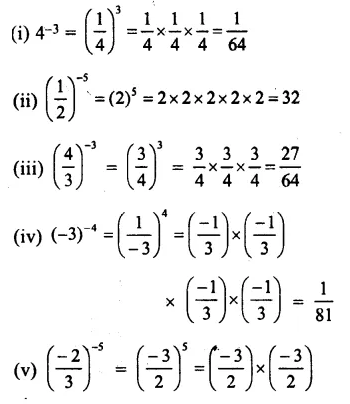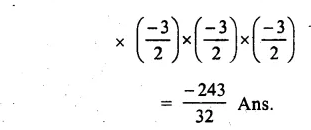Question 2.
Solution: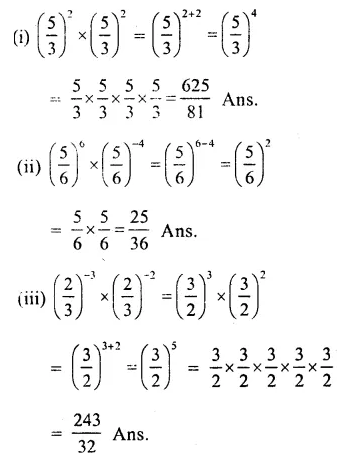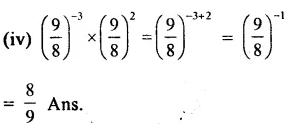Question 3.
Solution: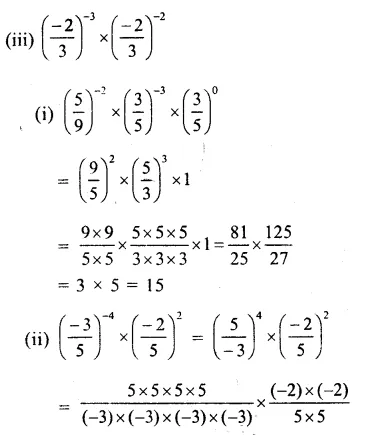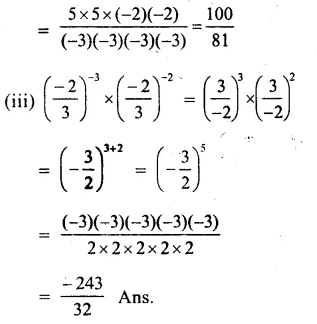Question 4.
Solution: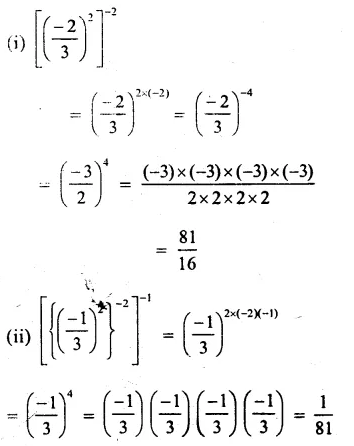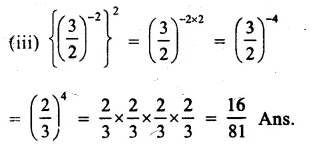Question 5.
Solution: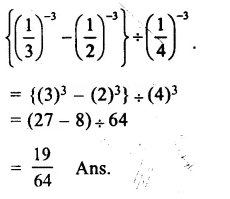Question 6.
Solution: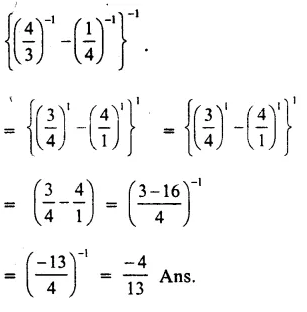Question 7.
Solution: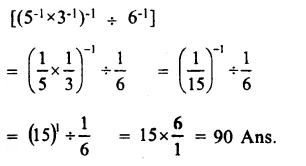Question 8.
Solution: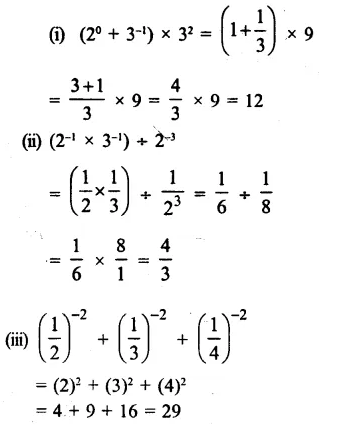Question 9.
Solution: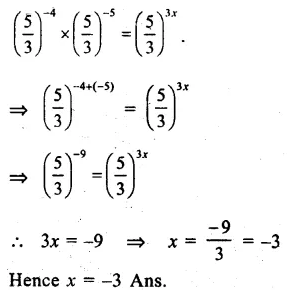Question 10.
Solution: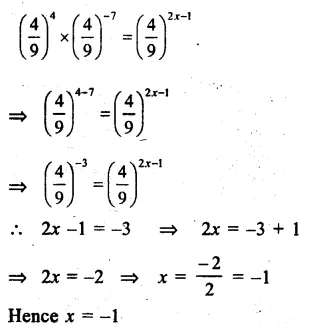Question 11.
Solution:
Let x be the required number which is multiplied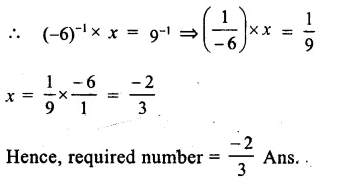Question 12.
Solution:
Let x be the required number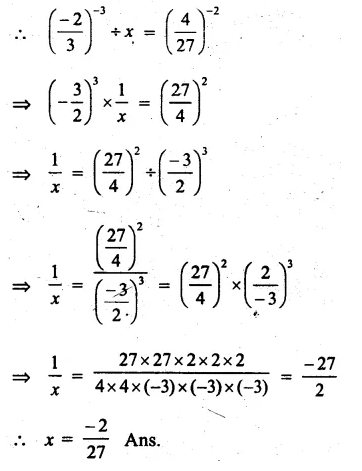Question 13.
Solution:
52x+1 ÷ 25 = 125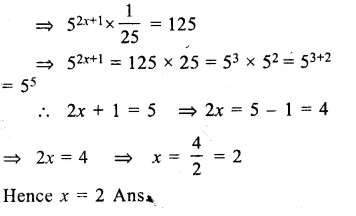Hope given RS Aggarwal Solutions Class 8 Chapter 2 Exponents Ex 2A are helpful to complete your math homework.

If you have any doubts, please comment below. Learn Insta try to provide online math tutoring for you.